﻿ 渐开线直齿轮机械功率损失和啮合效率预测 Meshing Efficiency and Mechanical Power Loss Prediction of Involute Spur Gear

Mechanical Engineering and Technology
Vol. 08  No. 03 ( 2019 ), Article ID: 30593 , 13 pages
10.12677/MET.2019.83022

Meshing Efficiency and Mechanical Power Loss Prediction of Involute Spur Gear

Yanfang Liu1,2, Xuelian Song1,2, Peng Dong1,2, Hongzhi Ji3, Gang Shao3, Qiang Liu3

1School of Transportation Science and Engineering, Beihang University, Beijing

2Beijing Key Laboratory for High Efficient Transmission and System Control of New Energy Resource Vehicle, Beijing

3No. 703 Research Institute of CSIC, Harbin Heilongjiang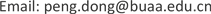Received: May 9th, 2019; accepted: May 24th, 2019; published: May 31st, 2019ABSTRACT

Understanding the mechanism of mechanical power loss occurring during gear meshing is a prerequisite for achieving high-efficiency design goals of gear transmission, and it is greatly affected by the lubrication characteristics during gear meshing. Based on the finite-line contact elastohydrodynamic lubrication theory, a transient elastohydrodynamic lubrication model of involute spur gear is established. The lubrication characteristics such as sliding/rolling speed, oil film pressure and oil film thickness at the gear meshing point are analyzed, and then the mechanical power loss prediction model of the involute spur gear is established. The mechanical power loss occurs during the meshing process of the spur gear is studied by the key parameters such as input torque, input speed and pressure angle, and the variation of the meshing efficiency is predicted. The simulation results show that the model established in this paper can effectively reveal the mechanism of the meshing efficiency of the involute spur gear, and find that the minimum meshing efficiency during the meshing process is the key factor determining the average meshing efficiency in the case where the maximum meshing efficiency level in the single-tooth meshing zone is generally high, which provides an effective reference for designing high efficiency involute spur gears.

Keywords:Elastohydrodynamic Lubrication (EHL), Involute Spur Gear, Meshing Efficiency

1北京航空航天大学交通科学与工程学院，北京

2新能源汽车高效动力传动与系统控制北京市重点实验室，北京

3中国船舶重工集团有限公司第七〇三研究所，黑龙江 哈尔滨Copyright © 2019 by authors and Hans Publishers Inc.1. 引言

2. 渐开线直齿轮瞬态弹流润滑模型

2.1. 直齿轮啮合模型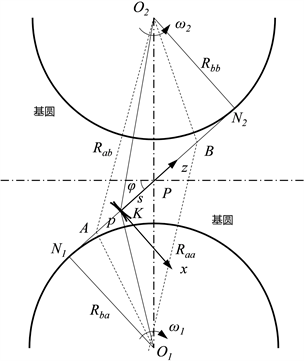Figure 1. Geometric contact model of spur gear

$\left\{\begin{array}{c}{R}_{a}={R}_{ba}\mathrm{tan}\phi +s\\ {R}_{b}={R}_{bb}\mathrm{tan}\phi -s\end{array}$ (1)

Rba——小齿轮的基圆半径；Rbb——大齿轮的基圆半径。

$\begin{array}{c}{u}_{a}={\omega }_{a}\left({R}_{ba}\mathrm{tan}\phi +s\right)\\ {u}_{b}={\omega }_{b}\left({R}_{bb}\mathrm{tan}\phi -s\right)\\ {\omega }_{a}{z}_{a}={\omega }_{b}{z}_{b}\end{array}$ (2)

${u}_{e}=\left({u}_{a}+{u}_{b}\right)/2$ (3)

${u}_{s}={u}_{a}-{u}_{b}$ (4)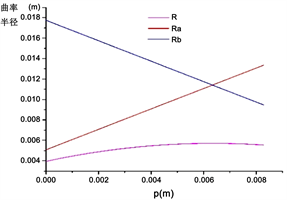Figure 2. Curvature radius of spur gear in meshing process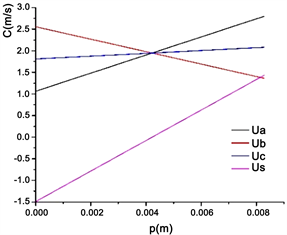Figure 3. Relevant speed parameters in spur gear meshing process

2.2. 瞬态润滑控制方程

$\frac{\partial }{\partial X}\left(\epsilon \frac{\partial P}{\partial X}\right)={C}_{u}\left(t\right)\frac{\partial }{\partial X}\left({\stackrel{¯}{\rho }}^{*}H\right)+\frac{\partial }{\partial \stackrel{¯}{t}}\left({\stackrel{¯}{\rho }}_{\epsilon }H\right)$ (5)

$P\left(t\right)=p\left(t\right)/{p}_{H}$

p——油膜压力

$\stackrel{¯}{t}=t{u}_{ep}/b$

t——时间

b——一半接触面长度

$X=x/b$

${\rho }^{*}=\left[{{\rho }^{\prime }}_{e}{\eta }_{e}\left({u}_{b}-{u}_{a}\right)+{\rho }_{e}{u}_{a}\right]/{u}_{e}$

${\stackrel{¯}{\rho }}_{e}={\rho }_{e}/{\rho }_{0}$

ρ——流体密度

η——流体粘度

${C}_{u}\left(t\right)={u}_{e}\left(t\right)/{u}_{ep}$

uep——节点处的滚动速度

$H\left(t\right)=h\left(t\right){R}_{0}/{b}^{2}$

R0——节点处等效曲率半径

$H\left(\stackrel{¯}{t}\right)={H}_{0}\left(\stackrel{¯}{t}\right)+\frac{{X}^{2}}{2{C}_{R}\left(\stackrel{¯}{t}\right)}-\frac{1}{\text{π}}{\int }_{{X}_{in}}^{{X}_{out}}P\left({X}^{\prime }\right)\mathrm{ln}|X-{X}^{\prime }|\text{d}{X}^{\prime }$ (6)

${C}_{R}\left(t\right)=R\left(t\right)/{R}_{0}$

CR(t)——综合曲率半径

Xin——润滑介质的入口坐标

Xout——润滑介质的出口坐标

${\int }_{{X}_{in}}^{{X}_{out}}P{\text{d}}_{X}=\frac{\text{π}}{2}{C}_{w}\left(t\right)$ (7)

${C}_{w}\left(t\right)=w\left(t\right)/{w}_{0}$

w0——节点处的单位载荷

$\left\{\begin{array}{c}P\left({X}_{in}\right)=0\\ P\left({X}_{out}\right)=0\\ P\ge 0,\left({X}_{in}

2.3. 润滑特性分析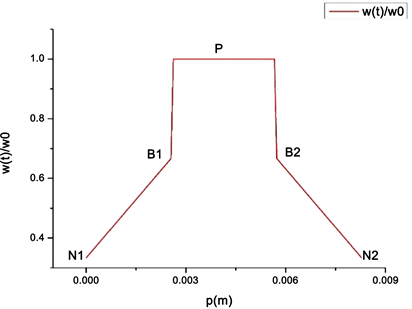Figure 4. Dimensionless load distribution map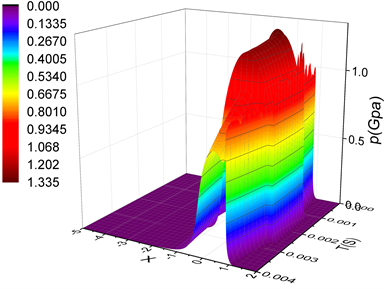Figure 5. Oil film pressure distribution mapFigure 6. Oil film thickness distribution map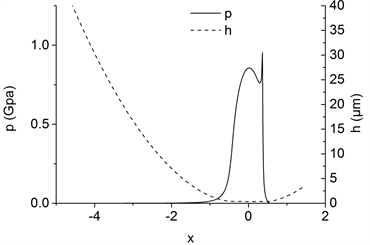(a) N1点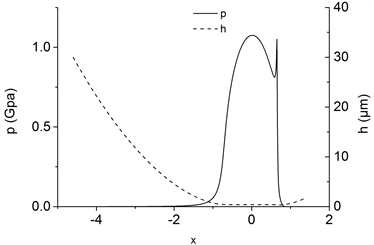(b) B1点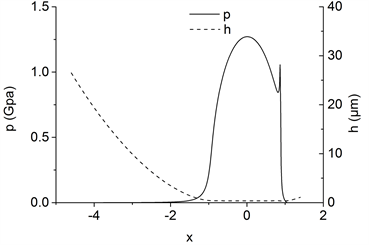(c) P点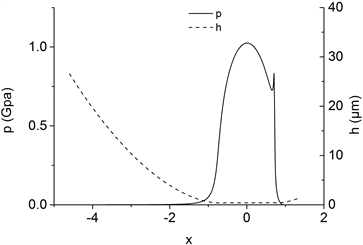(d) B2点(e) N2点

Figure 7. Oil film pressure and thickness distribution at basic meshing point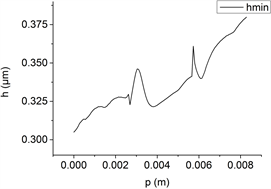(a) 最小油膜厚度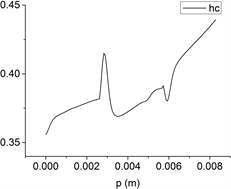(b) 中心油膜厚度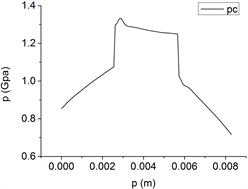(c) 油膜压力

Figure 8. Variation of oil film thickness and pressure with meshing line at central position

3. 直齿轮啮合效率预测模型

1) 摩擦因数取为平均值，由上述的热弹流润滑模型计算得出的平均速度来计算  。

2) 啮合过程中，齿轮表面受均等载荷。

${P}_{s}=f{F}_{n}{u}_{s}×{10}^{-3}$ (8)

f——啮合点处的摩擦系数；

Fn——法向齿面载荷；

us——瞬时滑动速度。

${P}_{r}=9{h}_{\mathrm{min}}{u}_{r}l×{10}^{-3}\mathrm{cos}\left(\phi \right)$ (9)

hmin——瞬时啮合点的最小油膜厚度；

ur——瞬时滚动速度；

l——齿宽；

φ——齿轮压力角。

$P=T\cdot n/9550$ (10)

T——齿轮组输入扭矩；

n——主动轮转速。

${\eta }_{g}={\left(P-{P}_{r}-P\right)}_{s}/P$ (11)

4. 啮合效率和机械效率损失分析

4.1. 不同转矩输入工况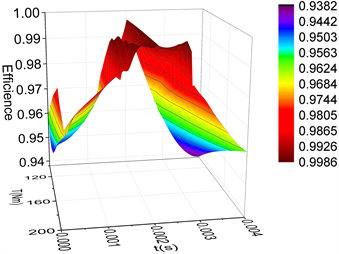(a) 啮合效率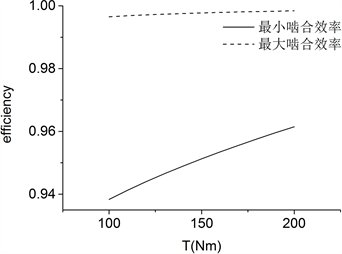(b) 啮合效率极值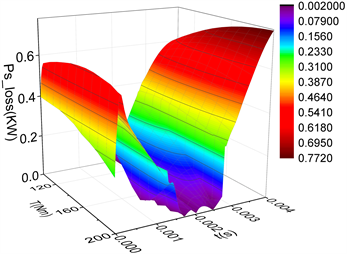(c) 滑动摩擦损失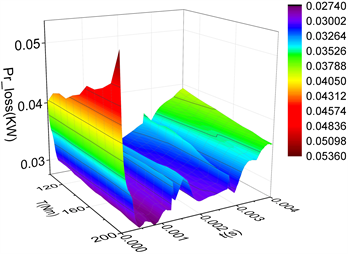(d) 滚动摩擦损失

Figure 9. Change of mechanical power loss and meshing efficiency of spur gears in meshing process under different input torques

4.2. 不同转速输入工况(a) 啮合效率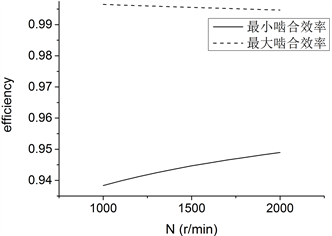(b) 啮合效率极值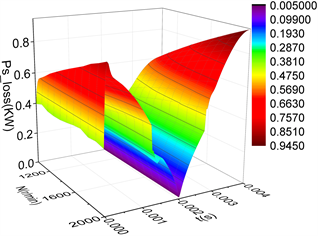(c) 滑动摩擦损失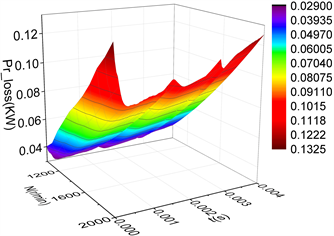(d) 滚动摩擦损失

Figure 10. Change of mechanical power loss and meshing efficiency of spur gears in meshing process at different input speed

4.3. 不同压力角对啮合效率的影响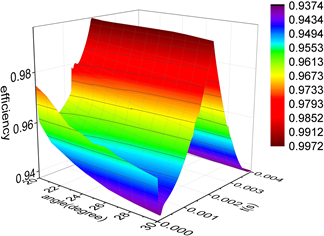(a) 啮合效率(b) 啮合效率极值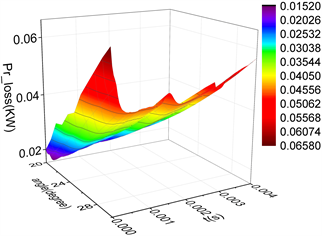(c) 滑动摩擦损失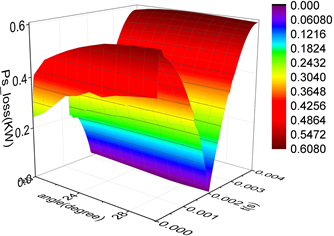(d) 滚动摩擦损失

Figure 11. Change of mechanical power loss and meshing efficiency of spur gears in meshing process at different pressure angles

5. 结论

1) 基于直齿轮啮合的几何特征建立了直齿轮啮合接触模型，可以计算任意啮合点处的曲率和速度；基于牛顿流体和润滑理论，建立了直齿轮瞬态弹流润滑模型，可以计算任意啮合点处的油膜压力和油膜厚度，与Hertz接触理论所得齿面压力不同，在润滑介质出口处存在第二压力峰，且同时存在油膜颈缩现象，揭示了直齿轮啮合中的弹流润滑状态。

2) 建立了直齿轮啮合效率模型，可以预测不同工况下的啮合效率及其变化规律，仿真结果发现有：低转矩、低转速和大压力角都会造成啮合平均效率偏低；单齿啮合区的最大啮合效率水平普遍较高的情况下，啮合过程中的最小啮合效率的大小是决定平均啮合效率高低的关键因素，其以滑动摩擦损失为主，且效率最低点往往发生在啮合过程中的啮入点等，为设计高效率直齿轮提供有效的参考依据。

Meshing Efficiency and Mechanical Power Loss Prediction of Involute Spur Gear[J]. 机械工程与技术, 2019, 08(03): 175-187. https://doi.org/10.12677/MET.2019.83022

1. 1. 周哲波. 弹流润滑状态下齿轮啮合效率的研究[J]. 机械设计, 2004, 21(12): 40-43.

2. 2. Li, S. and Kahraman, A. (2010) A Tran-sient Mixed Elastohydrodynamic Lubrication Model for Spur Gear Pairs. Journal of Tribology, 132, 011501. https://doi.org/10.1115/1.4000270

3. 3. 王文中, 操鸿, 胡纪滨. 渐开线斜齿轮非稳态弹流润滑数值模拟研究[J]. 摩擦学学报, 2011, 31(6): 604-609.

4. 4. Kolivand, M., Li, S. and Kahraman, A. (2010) Prediction of Mechanical Gear Mesh Efficiency of Hypoid Gear Pairs. Mechanism & Machine Theory, 45, 1568-1582. https://doi.org/10.1016/j.mechmachtheory.2010.06.015

5. 5. 王斌, 陈辛波. 混合润滑状态下渐开线直齿轮啮合效率分析[J]. 同济大学学报(自然科学版), 2014, 42(12): 1904-1911.

6. 6. 畅通, 王优强. 考虑粗糙度的直齿圆柱齿轮热混合润滑分析[J]. 润滑与密封, 2009, 34(4): 35-39.

7. 7. Martin, K.F. (1978) A Re-view of Friction Prediction in Gear Teeth. Wear, 49, 201-239. https://doi.org/10.1016/0043-1648(78)90088-1

8. 8. Hamrock, B.J. and Jacobson, B.O. (1981) Elastohydrodynamic Lubrication of Line Contacts. ASLE Transactions, 27, 275-287. https://doi.org/10.1080/05698198408981572

9. 9. Wang, C., Wang, S.R. and Wang, G.Q. (2018) A Method for Calculating Gear Meshing Efficiency by Measured Data from Gear Test Machine. Measurement, 119, 97-101. https://doi.org/10.1016/j.measurement.2018.01.031

10. 10. Prakash, D.P. and Patelan, J.M. (2012) Experimental Investigation of Power Losses in Manual Transmission Gear Box. International Journal of Applied Research in Mechanical En-gineering, 2, 1-5.

11. 11. Moorhead, M.D. (2007) Experimental Investigation of Spur Gear Efficiency and the Development of a Helical Gear Efficiency Test Machine. The Ohio State University, Ohio.